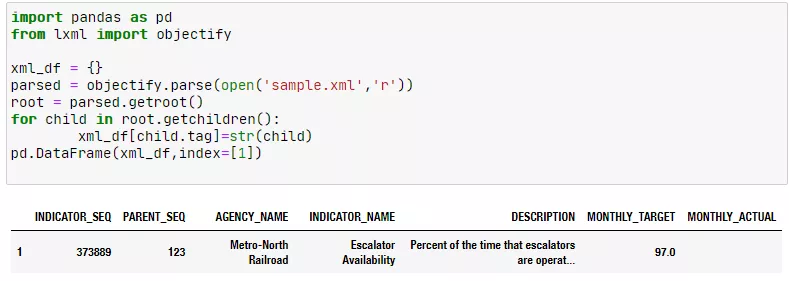# Python 数据读取、存储与⽂件格式

## 1 概述

pandas内置了10 余种数据源读取函数和对应的数据写人函数。常见的数据源有3种，分别是数据库数据、文本文件(包括一般文本文件和CSV文件)和Excel文件。掌握这3种数据源读取方法，便能够完成80%左右的数据读取工作。

## 2 数据读取

``````import pandas as pd
import numpy as np

np.savetxt('test2.csv',np.random.rand(10000,3),delimiter=',')``````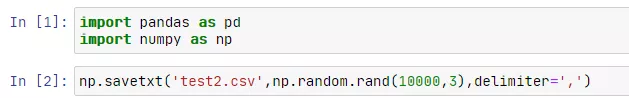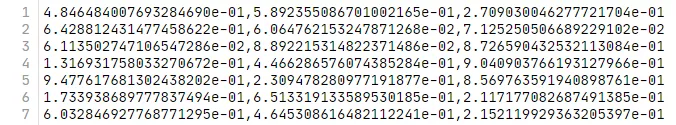……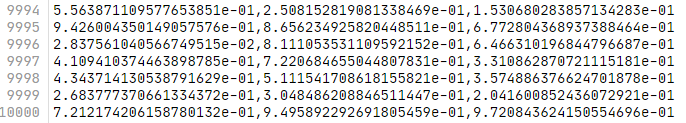``pd.read_csv('test2.csv',names=[1,2,3])``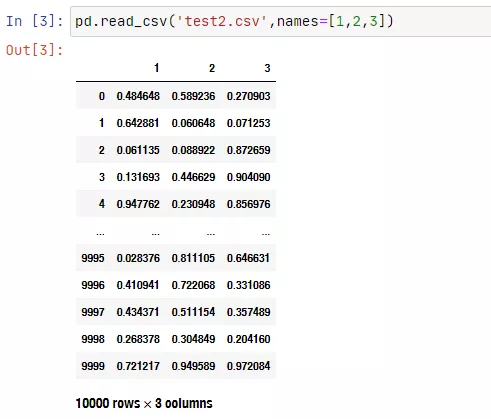``pd.options.display.max_rows #output：60``

``````pd.options.display.max_rows = 5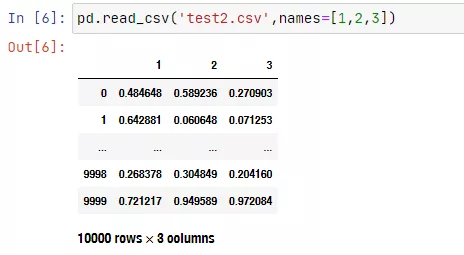``pd.read_csv('test2.csv',names=[1,2,3],nrows=5)``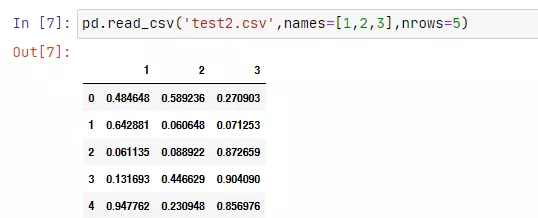``````df = pd.read_csv('test2.csv',names=[1,2,3],nrows=5)
df.to_csv('test3.csv')``````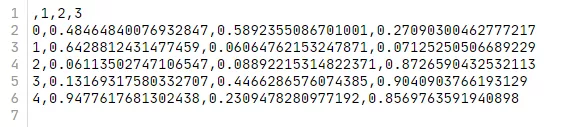``````Signature:
df.to_csv(    path_or_buf: 'Optional[FilePathOrBuffer]' = None,    sep: 'str' = ',',    na_rep: 'str' = '',    float_format: 'Optional[str]' = None,    columns: 'Optional[Sequence[Label]]' = None,    header: 'Union[bool_t, List[str]]' = True,    index: 'bool_t' = True,    index_label: 'Optional[IndexLabel]' = None,    mode: 'str' = 'w',    encoding: 'Optional[str]' = None,    compression: 'CompressionOptions' = 'infer',    quoting: 'Optional[int]' = None,    quotechar: 'str' = '"',    line_terminator: 'Optional[str]' = None,    chunksize: 'Optional[int]' = None,    date_format: 'Optional[str]' = None,    doublequote: 'bool_t' = True,    escapechar: 'Optional[str]' = None,    decimal: 'str' = '.',    errors: 'str' = 'strict',    storage_options: 'StorageOptions' = None,) -> 'Optional[str]'
Docstring:
Write object to a comma-separated values (csv) file.``````

``````import csv

print(csv.__all__)``````

``````['QUOTE_MINIMAL', 'QUOTE_ALL', 'QUOTE_NONNUMERIC', 'QUOTE_NONE',
'Error', 'Dialect', '__doc__', 'excel', 'excel_tab', 'field_size_limit',
'reader', 'writer', 'register_dialect', 'get_dialect', 'list_dialects',
'Sniffer', 'unregister_dialect', '__version__', 'DictReader', 'DictWriter', 'unix_dialect']``````

``````fp = open('test.csv','r')
for line in csv_reader:
print(line)
fp.close()``````

``````['a', 'b', 'c', 'd', 'message']
['1', '2', '3', '4', 'hello']
['5', '6', '7', '8', '']
['9', '10', '11', '12', 'foo']``````

csv.writer可以向文件中写入数据。

``````csv_writer = csv.writer(fileobj [, dialect='excel']
[optional keyword args])
for row in sequence:
csv_writer.writerow(row)
[or]
csv_writer = csv.writer(fileobj [, dialect='excel']
[optional keyword args])
csv_writer.writerows(rows)``````
``````class my_dialect(csv.Dialect):
lineterminator = '\n'
delimiter = ','
quotechar = '"'
quoting = csv.QUOTE_MINIMAL
csv_list = []
fp = open('test.csv','r')
for line in csv_reader:
csv_list.append(line)
fp.close()
fp2 = open('test_2.csv','w')
csv_writer = csv.writer(fp2,dialect=my_dialect)
for line in csv_list[:3]:
csv_writer.writerow(line)
fp2.close()``````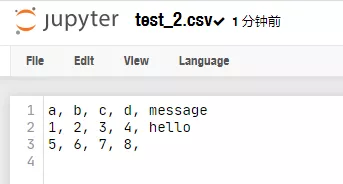csv写操作默认换行符是‘\r\n’，直接默认写入会出现空行的效果。

``````csv_writer = csv.writer(fp2)
for line in csv_list[:3]:
csv_writer.writerow(line)``````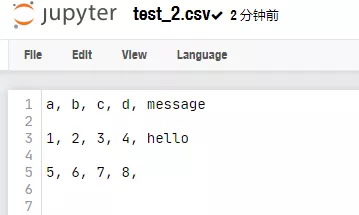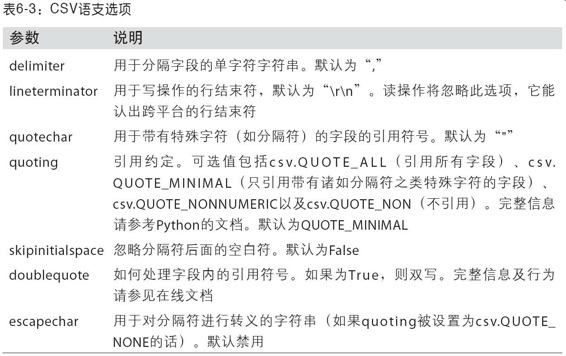JSON数据，JavaScript Object Notation的简称）已经成为通过HTTP请求在Web浏览器和其他应⽤程序之间发送数据的标准格式之⼀。它是⼀种⽐表格型⽂本格式（如CSV）灵活得多的数据格式。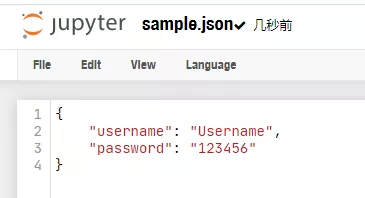``````import pandas as pd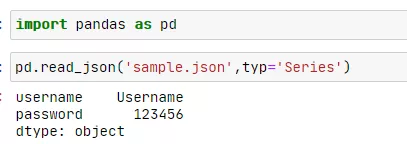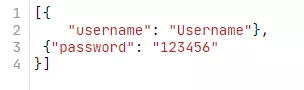``pd.read_json('sample.json')``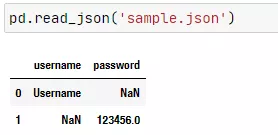``pd.read_json?``

DataFrame对象的to_json方法可以写入文件。

``````import numpy as np

df = pd.DataFrame(np.random.rand(3,3),columns=['A','B','C'])
df.to_json('sample2.json')``````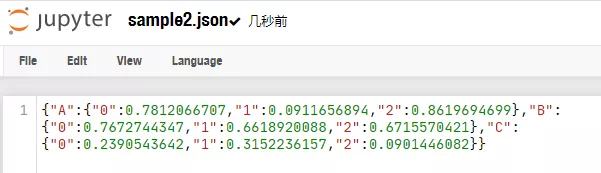``df.to_json?``

XML和HTML：Web信息收集.

lxml和BeautifulSoup虽然有使用过，但是一直没有说明，作为非标准模块，使用前需要安装。

``````pip install beautifulsoup4
pip install lxml``````

``pd.read_html('12306.html')``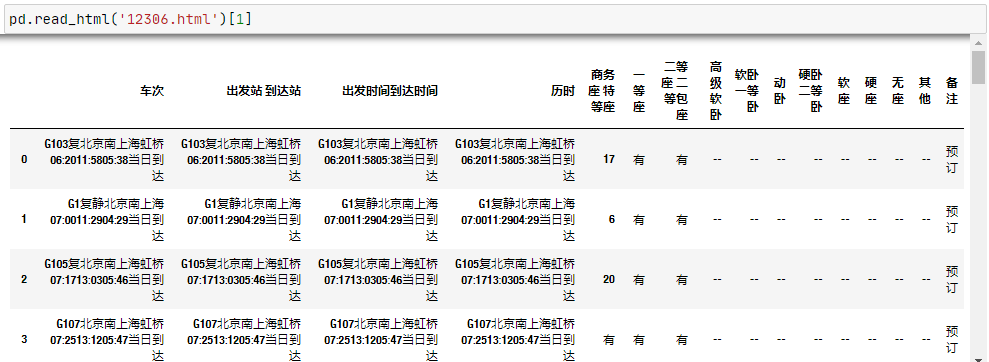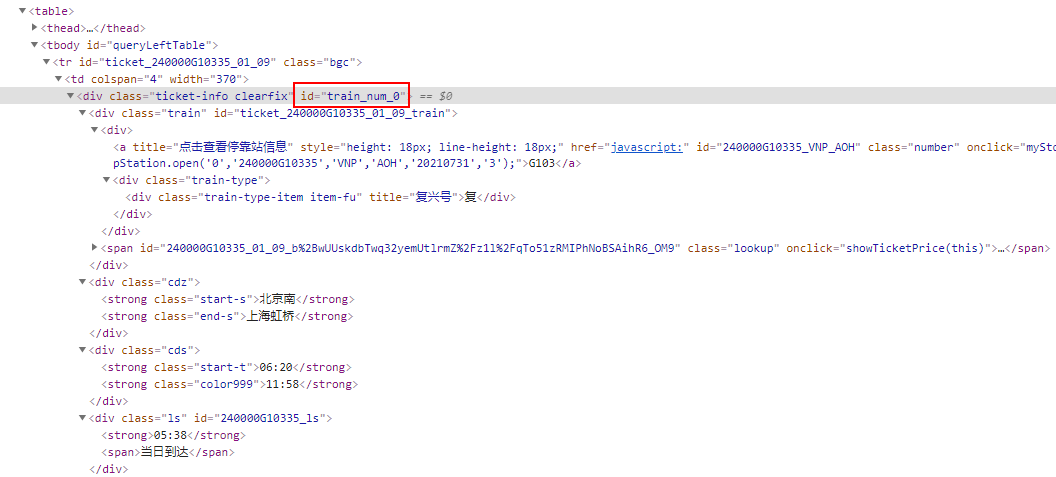``````from lxml import etree

fp = open('12306.html','r',encoding='utf-8')
tree = etree.HTML(fp_content)
for i in range(20):
train_msg = tree.xpath(f'//*[@id="train_num_{i}"]//text()')
print(train_msg)
fp.close()``````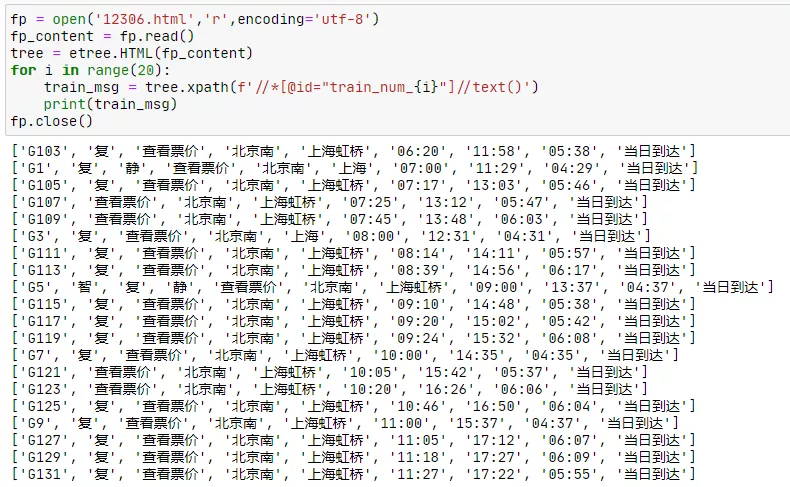XML（Extensible Markup Language）是另⼀种常⻅的⽀持分层、嵌套数据以及元数据的结构化数据格式。XML和HTML的结构很相似，但XML更为通⽤。如果熟悉C#或者其他语言，XML文件通常也可以用来作为配置文件，用于给用户自定义信息。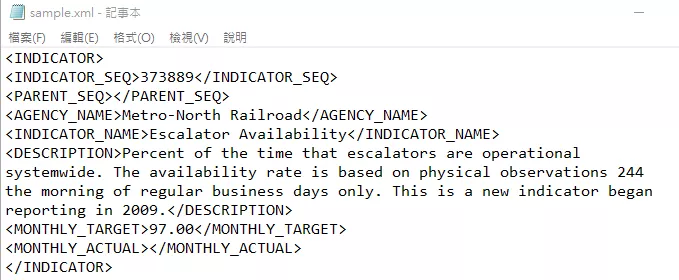``````import pandas as pd
from lxml import objectify

xml_df = {}
parsed = objectify.parse(open('sample.xml','r'))
root = parsed.getroot()
for child in root.getchildren():
xml_df[child.tag]=str(child)
pd.DataFrame(xml_df,index=)``````